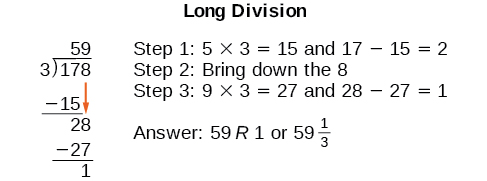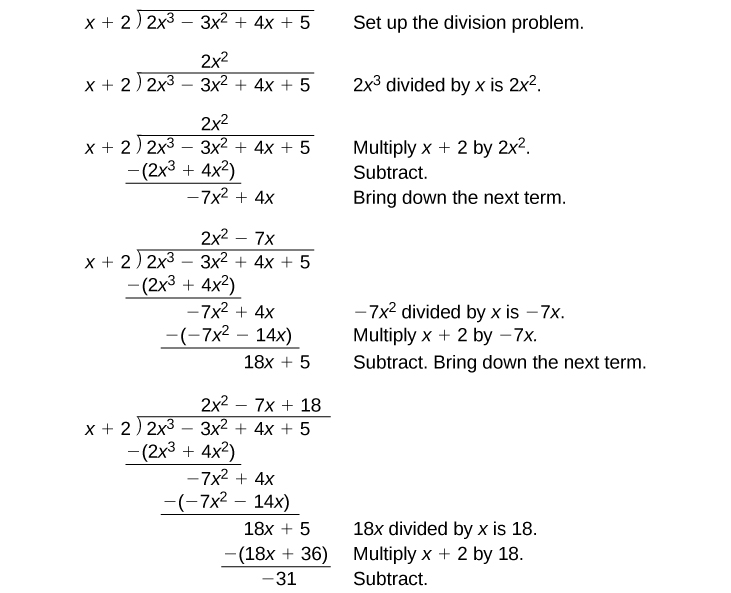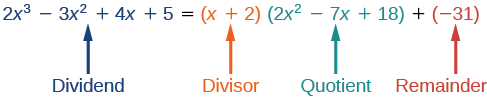5.4 Dividing polynomials

 Page 1 / 6
In this section, you will:
• Use long division to divide polynomials.
• Use synthetic division to divide polynomials.Lincoln Memorial, Washington, D.C. (credit: Ron Cogswell, Flickr)

The exterior of the Lincoln Memorial in Washington, D.C., is a large rectangular solid with length 61.5 meters (m), width 40 m, and height 30 m. National Park Service. "Lincoln Memorial Building Statistics." http://www.nps.gov/linc/historyculture/lincoln-memorial-building-statistics.htm. Accessed 4/3/2014 We can easily find the volume using elementary geometry.

$\begin{array}{ccc}\hfill V& =& l\cdot w\cdot h\hfill \\ & =& 61.5\cdot 40\cdot 30\hfill \\ & =& 73,800\hfill \end{array}$

So the volume is 73,800 cubic meters $\text{\hspace{0.17em}}\left(\text{m}³\right).\text{\hspace{0.17em}}$ Suppose we knew the volume, length, and width. We could divide to find the height.

$\begin{array}{ccc}\hfill h& =& \frac{V}{l\cdot w}\hfill \\ & =& \frac{73,800}{61.5\cdot 40}\hfill \\ & =& 30\hfill \end{array}$

As we can confirm from the dimensions above, the height is 30 m. We can use similar methods to find any of the missing dimensions. We can also use the same method if any, or all, of the measurements contain variable expressions. For example, suppose the volume of a rectangular solid is given by the polynomial $\text{\hspace{0.17em}}3{x}^{4}-3{x}^{3}-33{x}^{2}+54x.\text{\hspace{0.17em}}$ The length of the solid is given by $\text{\hspace{0.17em}}3x;\text{\hspace{0.17em}}$ the width is given by $\text{\hspace{0.17em}}x-2.\text{\hspace{0.17em}}$ To find the height of the solid, we can use polynomial division, which is the focus of this section.

Using long division to divide polynomials

We are familiar with the long division algorithm for ordinary arithmetic. We begin by dividing into the digits of the dividend that have the greatest place value. We divide, multiply, subtract, include the digit in the next place value position, and repeat. For example, let’s divide 178 by 3 using long division.Another way to look at the solution is as a sum of parts. This should look familiar, since it is the same method used to check division in elementary arithmetic.

We call this the Division Algorithm and will discuss it more formally after looking at an example.

Division of polynomials that contain more than one term has similarities to long division of whole numbers. We can write a polynomial dividend as the product of the divisor and the quotient added to the remainder. The terms of the polynomial division correspond to the digits (and place values) of the whole number division. This method allows us to divide two polynomials. For example, if we were to divide $\text{\hspace{0.17em}}2{x}^{3}-3{x}^{2}+4x+5\text{\hspace{0.17em}}$ by $\text{\hspace{0.17em}}x+2\text{\hspace{0.17em}}$ using the long division algorithm, it would look like this:We have found

$\frac{2{x}^{3}-3{x}^{2}+4x+5}{x+2}=2{x}^{2}-7x+18-\frac{31}{x+2}$

or

$\frac{2{x}^{3}-3{x}^{2}+4x+5}{x+2}=\left(x+2\right)\left(2{x}^{2}-7x+18\right)-31$

We can identify the dividend , the divisor , the quotient , and the remainder .Writing the result in this manner illustrates the Division Algorithm.

The division algorithm

The Division Algorithm    states that, given a polynomial dividend $\text{\hspace{0.17em}}f\left(x\right)\text{\hspace{0.17em}}$ and a non-zero polynomial divisor $\text{\hspace{0.17em}}d\left(x\right)\text{\hspace{0.17em}}$ where the degree of $\text{\hspace{0.17em}}d\left(x\right)\text{\hspace{0.17em}}$ is less than or equal to the degree of $\text{\hspace{0.17em}}f\left(x\right)$ , there exist unique polynomials $\text{\hspace{0.17em}}q\left(x\right)\text{\hspace{0.17em}}$ and $\text{\hspace{0.17em}}r\left(x\right)\text{\hspace{0.17em}}$ such that

$f\left(x\right)=d\left(x\right)q\left(x\right)+r\left(x\right)$

$q\left(x\right)\text{\hspace{0.17em}}$ is the quotient and $\text{\hspace{0.17em}}r\left(x\right)\text{\hspace{0.17em}}$ is the remainder. The remainder is either equal to zero or has degree strictly less than $\text{\hspace{0.17em}}d\left(x\right).\text{\hspace{0.17em}}$

If $\text{\hspace{0.17em}}r\left(x\right)=0,\text{\hspace{0.17em}}$ then $\text{\hspace{0.17em}}d\left(x\right)\text{\hspace{0.17em}}$ divides evenly into $\text{\hspace{0.17em}}f\left(x\right).\text{\hspace{0.17em}}$ This means that, in this case, both $\text{\hspace{0.17em}}d\left(x\right)\text{\hspace{0.17em}}$ and $\text{\hspace{0.17em}}q\left(x\right)\text{\hspace{0.17em}}$ are factors of $\text{\hspace{0.17em}}f\left(x\right).\text{\hspace{0.17em}}$

sin^4+sin^2=1, prove that tan^2-tan^4+1=0
what is the formula used for this question? "Jamal wants to save \$54,000 for a down payment on a home. How much will he need to invest in an account with 8.2% APR, compounding daily, in order to reach his goal in 5 years?"
i don't need help solving it I just need a memory jogger please.
Kuz
A = P(1 + r/n) ^rt
Dale
how to solve an expression when equal to zero
its a very simple
Kavita
gave your expression then i solve
Kavita
Hy guys, I have a problem when it comes on solving equations and expressions, can you help me 😭😭
Thuli
Tomorrow its an revision on factorising and Simplifying...
Thuli
ok sent the quiz
kurash
send
Kavita
Hi
Masum
What is the value of log-1
Masum
the value of log1=0
Kavita
Log(-1)
Masum
What is the value of i^i
Masum
log -1 is 1.36
kurash
No
Masum
no I m right
Kavita
No sister.
Masum
no I m right
Kavita
tan20°×tan30°×tan45°×tan50°×tan60°×tan70°
jaldi batao
Joju
Find the value of x between 0degree and 360 degree which satisfy the equation 3sinx =tanx
what is sine?
what is the standard form of 1
1×10^0
Akugry
Evalute exponential functions
30
Shani
The sides of a triangle are three consecutive natural number numbers and it's largest angle is twice the smallest one. determine the sides of a triangle
Will be with you shortly
Inkoom
3, 4, 5 principle from geo? sounds like a 90 and 2 45's to me that my answer
Neese
answer is 2, 3, 4
Gaurav
prove that [a+b, b+c, c+a]= 2[a b c]
can't prove
Akugry
i can prove [a+b+b+c+c+a]=2[a+b+c]
this is simple
Akugry
hi
Stormzy
x exposant 4 + 4 x exposant 3 + 8 exposant 2 + 4 x + 1 = 0
x exposent4+4x exposent3+8x exposent2+4x+1=0
HERVE
How can I solve for a domain and a codomains in a given function?
ranges
EDWIN
Thank you I mean range sir.
Oliver
proof for set theory
don't you know?
Inkoom
find to nearest one decimal place of centimeter the length of an arc of circle of radius length 12.5cm and subtending of centeral angle 1.6rad
factoring polynomialByBy Naveen TomarByBy